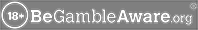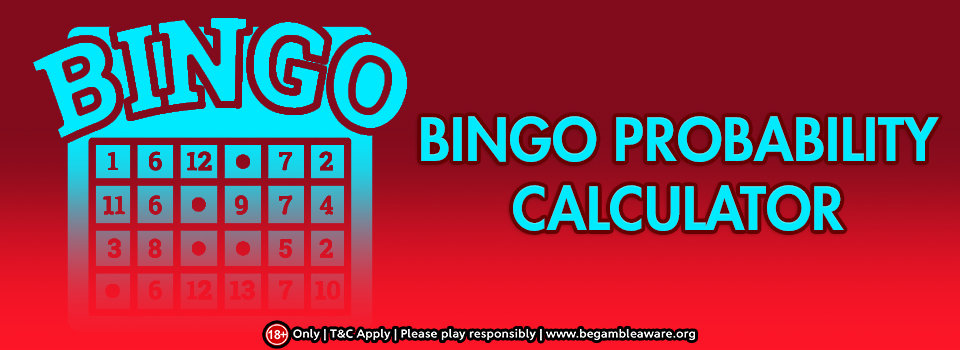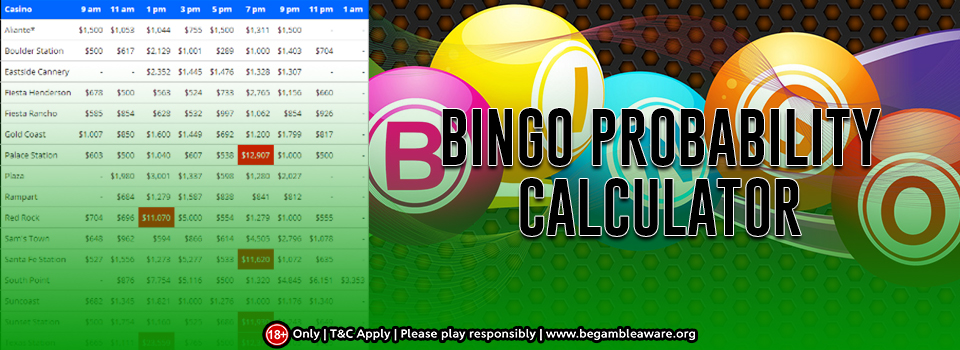# Bingo Probability Calculator

Bingo statistics can be calculated by ascertaining the Single Board Cumulative Probability of getting Bingo before the “Nth” number. These numbers are listed in the statistics table given below in the third column. You can easily calculate that with the help of a Bingo probability calculator.

The first step is to learn how to calculate the entries in the columns. The Bingo probability calculator can calculate the probability of getting Bingo in the case of any Nth number. This can be calculated for any number of boards like 1, 50 or any other. The probability of Bingo is calculated by ascertaining the  cumulative probability of the Nth number and the N-1 number and subtracting them.

Example – With a single board, the probability of winning a Bingo while the 20th number is called is equal to the difference in the cumulative probability of 20 numbers (0.022874) and the cumulative probability of 19 numbers (0.017993). The difference between 0.022874 and 0.017993 is 0.004881, the probability which is visible on the Bingo probability calculator.

Example – In a game of 50 boards, when the 20th number is called, what is the probability of getting a Bingo for at least one of the boards?

This is the difference between 0.685574 and 0.596608 which are the cumulative probability of the 20 and 19 numbers respectively. 0.088966 is the result and the Bingo probability.

## How to calculate the Column 5 data

The Cumulative probability can be easily calculated across different boards once the probability is calculated for the single board. If you assume “K” equal the total number of boards, and let Cp[N] be the single board Cumulative Probability after calling out N numbers, then the “K” boards Cumulative Probability after calling out N numbers is:

1 – ((1 – Cp[N])^K)    (^ is the exponential function )## Calculating the single board cumulative probability values

Until now, you have only been doing easy calculations using the Cumulative Probabilities for a single board. But have you thought about how you reach these numbers? Finding out these numbers requires some great calculation skills, or you could use a Bingo probability calculator.

The Single Board Cumulative probability for at least one Bingo game, where “N” numbers have been called can be calculated by summating the probabilities of the following outcomes:

Considering N numbers, when 4 of them are on the card and they form a Bingo

Considering N numbers, when 5 of them are on the card and they form a Bingo

Considering N numbers, when 6 of them are on the card and they form a Bingo

Considering N numbers, when K of them are on the card and they form a Bingo, etc

For each of the above outcomes, you are required to calculate, in case N numbers have been called, the probability of getting K matches. It is also important that you ascertain the probability of at least one Bingo when “K” numbers have been marked.

Now you calculate the probability of getting at least 1 Bingo

You know that you have “K” hits on the Bingo Card

(Warning: If you don’t like coding and playing around with  “C” or “C++” languages, you may not like the rest. Use a Bingo probability calculator in this case)

The basic idea is that there are 25 cells on a Bingo card that have been divided into a 5 x 5 square. The space in the center is vacant, which leaves you 24 spaces that may or may not match in the course of the play.

This leaves 2^24 = 16,777,216 total patterns. All these patterns may or may not have a Bingo. But all of these outcomes have an equal probability. For each of these outcomes, you will need to count how many spaces are vacant and how many are taken to see if the pattern contains a Bingo, or you could just use a Bingo probability calculator.